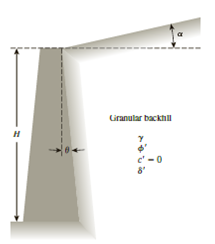Chapter 14, Problem 14.7PPrinciples of Geotechnical Enginee...

9th Edition
Braja M. Das + 1 other
ISBN: 9781305970939

Solutions

Chapter
SectionPrinciples of Geotechnical Enginee...

9th Edition
Braja M. Das + 1 other
ISBN: 9781305970939
Textbook Problem

Refer to the retaining wall shown in Figure 14.19. Given: θ = 10°, α = 0, γ = 122 lb/ft3, ϕ ′ = 35 ° , δ ′ = 21 ° , and H = 18 ft. Estimate the passive force, Pp, per unit length of the wall using Zhu and Qian’s (2000) method of triangular slices.Figure 14.19

To determine

Find the passive force Pp per unit length of the wall using Zhu and Qian’s method of triangular slices.

Explanation

Given information:

The height (H) of the retaining wall is 18 ft.

The soil friction angle ϕ is 35°.

The angle of wall friction δ is 21°.

The given angle θ value is 10°.

The unit weight γ of the granular backfill is 122lb/ft3.

Calculation:

Calculate passive force Pp per unit length of the wall using Zhu and Qian’s method of triangular slices.

Pp=12KpγH2 (1)

Here, Kp is the passive earth pressure coefficient.

Refer Table (14.4) “Variation of Kp=Kp(δ=0) and Figure 14.3a” in the text book.

Take the passive earth pressure coefficient Kp=Kp(δ=0) for the soil having friction angle of 35° and the angle of 10° as 2.97.

Determine the ratio of angle of wall friction to the soil friction angle.

δϕ=21°35°=0

Still sussing out bartleby?

Check out a sample textbook solution.

See a sample solution

The Solution to Your Study Problems

Bartleby provides explanations to thousands of textbook problems written by our experts, many with advanced degrees!

Get Started

What is data independence, and why is it lacking in file systems?

Database Systems: Design, Implementation, & Management

Define each of the following terms: a. data b. field c. record d. file

Database Systems: Design, Implementation, & Management

List and describe the three control strategies proposed for IDPSs.

Principles of Information Security (MindTap Course List)

What is ICANN and what role does it play?

Fundamentals of Information Systems

Define the term, spoofing. How can you tell if an email is spoofed?

Enhanced Discovering Computers 2017 (Shelly Cashman Series) (MindTap Course List)

Why must welding cables never be tied to scaffolding or ladders?

Welding: Principles and Applications (MindTap Course List)